# UNIT-III.pdf

29 May 2023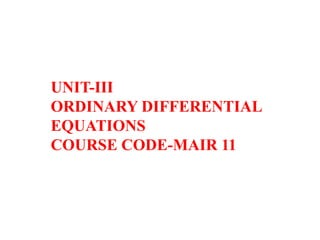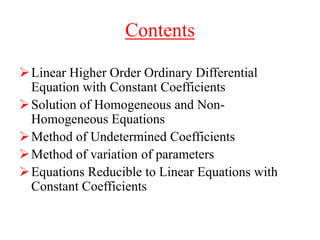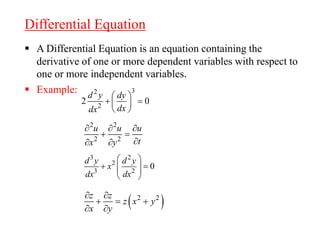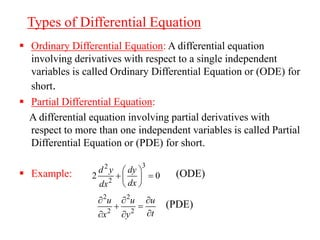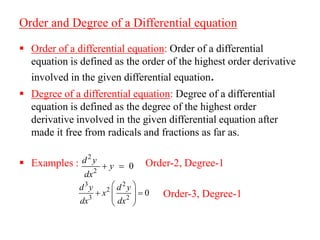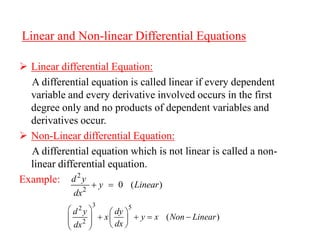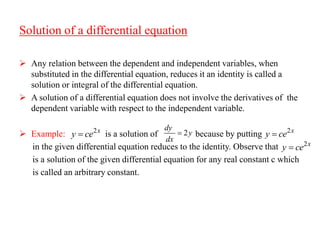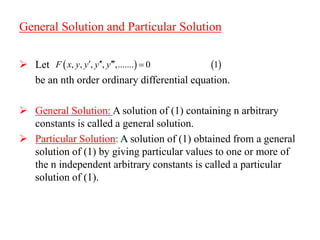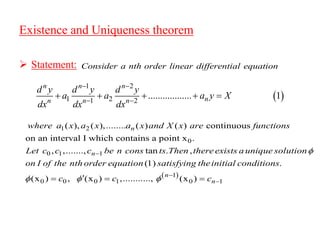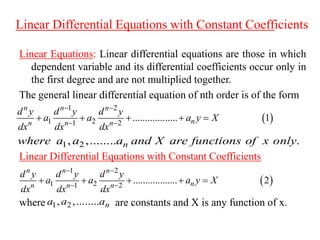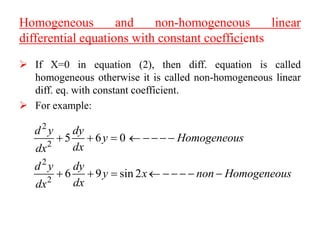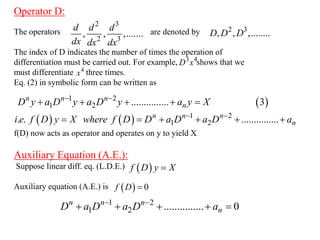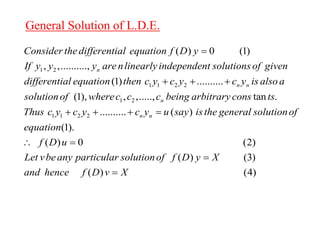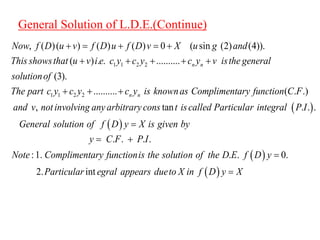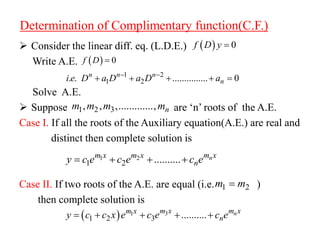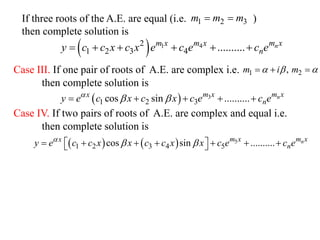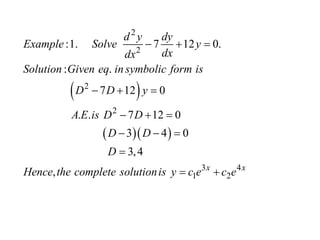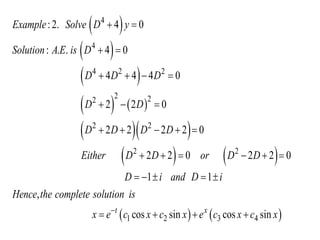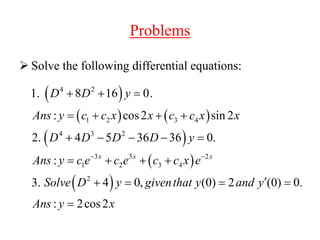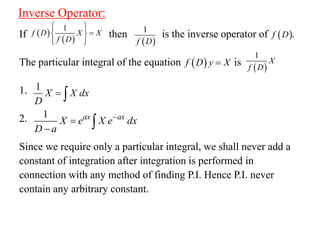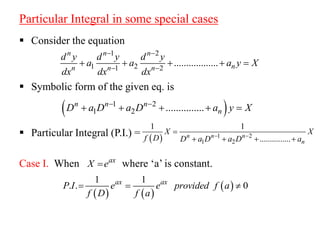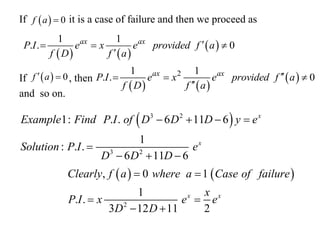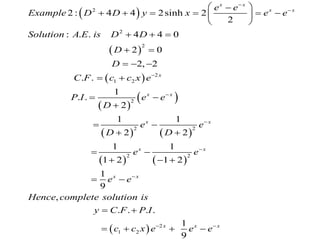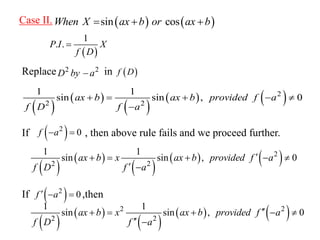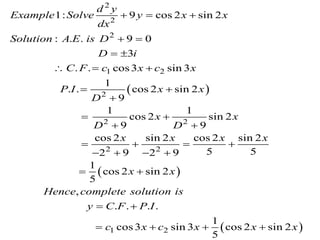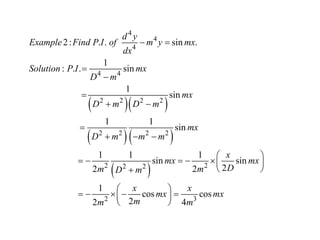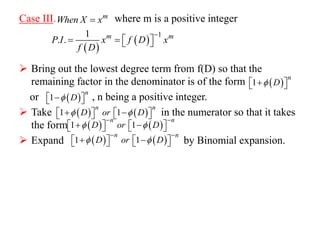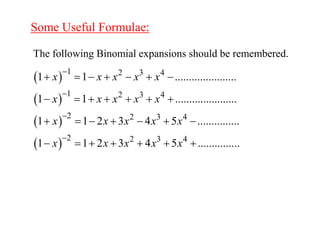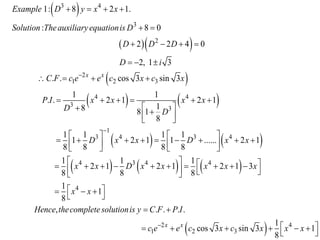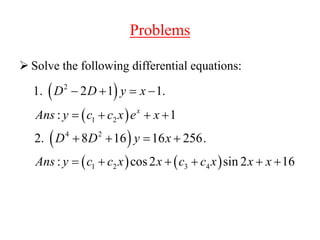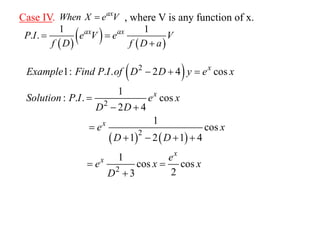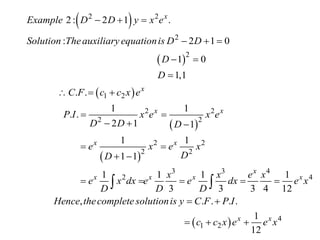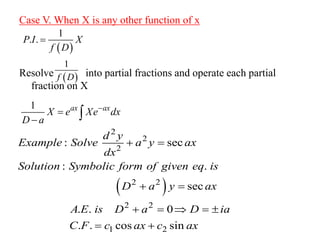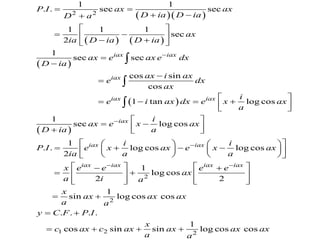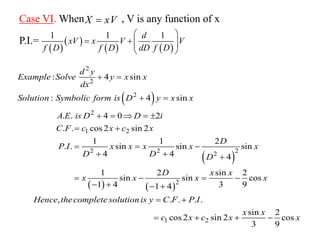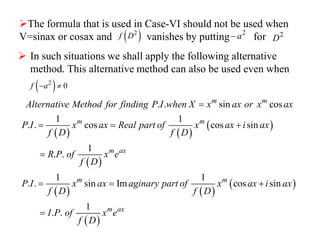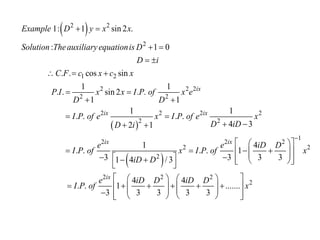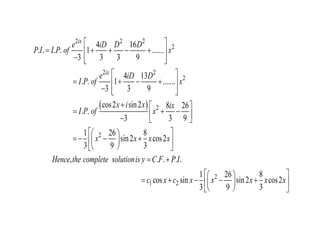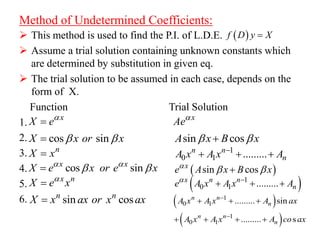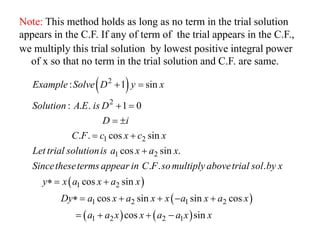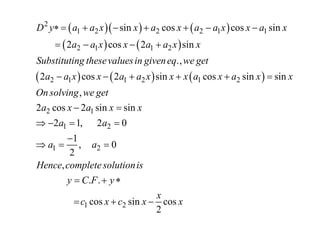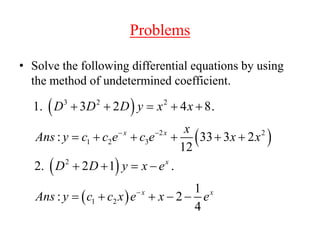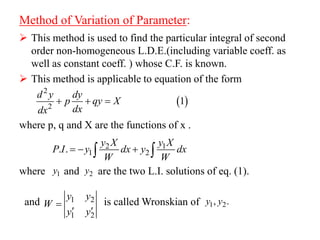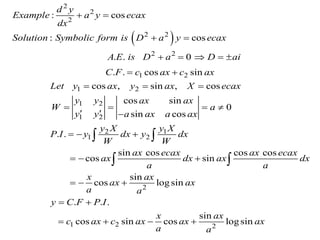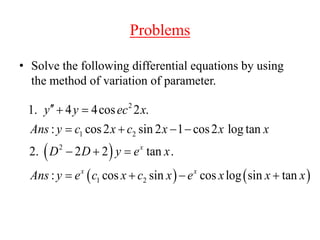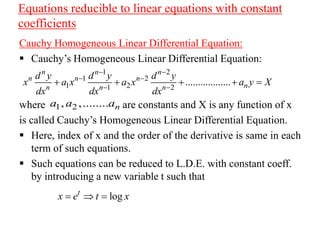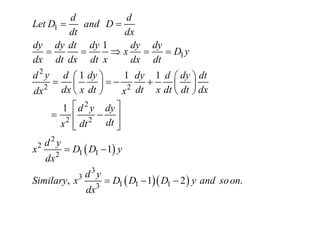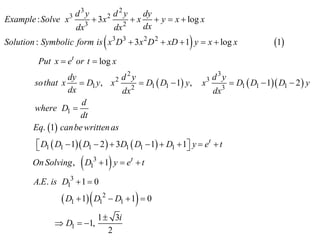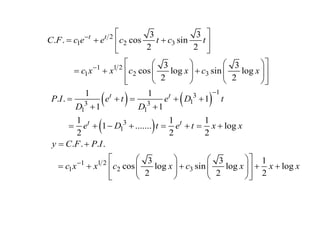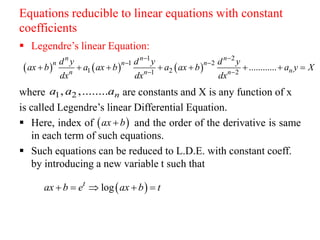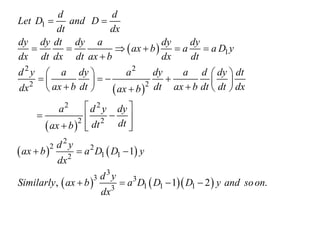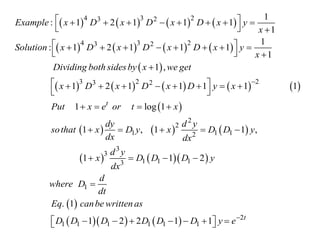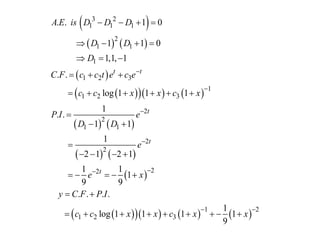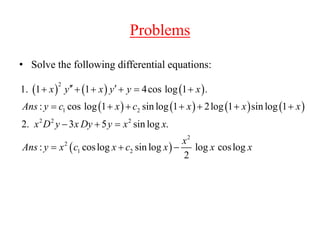1 sur 54

### UNIT-III.pdf

• 1. UNIT-III ORDINARY DIFFERENTIAL EQUATIONS COURSE CODE-MAIR 11
• 2. Contents Linear Higher Order Ordinary Differential Equation with Constant Coefficients Solution of Homogeneous and Non- Homogeneous Equations Method of Undetermined Coefficients Method of variation of parameters Equations Reducible to Linear Equations with Constant Coefficients
• 3. Differential Equation  A Differential Equation is an equation containing the derivative of one or more dependent variables with respect to one or more independent variables.  Example: 3 2 2 2 0 d y dy dx dx         2 2 2 2 u u u t x y         3 2 2 3 2 0 d y d y x dx dx             2 2 z z z x y x y       
• 4. Types of Differential Equation  Ordinary Differential Equation: A differential equation involving derivatives with respect to a single independent variables is called Ordinary Differential Equation or (ODE) for short.  Partial Differential Equation: A differential equation involving partial derivatives with respect to more than one independent variables is called Partial Differential Equation or (PDE) for short.  Example: (ODE) (PDE) 3 2 2 2 0 d y dy dx dx         2 2 2 2 u u u t x y        
• 5. Order and Degree of a Differential equation  Order of a differential equation: Order of a differential equation is defined as the order of the highest order derivative involved in the given differential equation.  Degree of a differential equation: Degree of a differential equation is defined as the degree of the highest order derivative involved in the given differential equation after made it free from radicals and fractions as far as.  Examples : Order-2, Degree-1 Order-3, Degree-1 2 2 0 d y y dx   3 2 2 3 2 0 d y d y x dx dx          
• 6. Linear and Non-linear Differential Equations  Linear differential Equation: A differential equation is called linear if every dependent variable and every derivative involved occurs in the first degree only and no products of dependent variables and derivatives occur.  Non-Linear differential Equation: A differential equation which is not linear is called a non- linear differential equation. Example: 3 5 2 2 ( ) d y dy x y x Non Linear dx dx                   2 2 0 ( ) d y y Linear dx  
• 7. Solution of a differential equation  Any relation between the dependent and independent variables, when substituted in the differential equation, reduces it an identity is called a solution or integral of the differential equation.  A solution of a differential equation does not involve the derivatives of the dependent variable with respect to the independent variable.  Example: is a solution of because by putting in the given differential equation reduces to the identity. Observe that is a solution of the given differential equation for any real constant c which is called an arbitrary constant. 2x y ce  2 dy y dx  2x y ce  2x y ce 
• 8. General Solution and Particular Solution  Let be an nth order ordinary differential equation.  General Solution: A solution of (1) containing n arbitrary constants is called a general solution.  Particular Solution: A solution of (1) obtained from a general solution of (1) by giving particular values to one or more of the n independent arbitrary constants is called a particular solution of (1).     , , , , ,....... 0 1 F x y y y y    
• 9. Existence and Uniqueness theorem  Statement:   1 2 1 2 1 2 .................. 1 n n n n n n n d y d y d y a a a y X dx dx dx          1 0 0 1 1 2 ( ) continuous on an interval I which contains a point x . , ,......., tan ( ), ( ),........ ( ( ) . , n n where and X x are functions Let c c c be n cons ts Then thereexists a unique solution on I of the nth order equ a x a x a x ation     1 0 0 0 1 0 1 1) . (x ) , (x ) ,..........., (x ) n n satisfying theinitial conditions c c c          Consider a nth order linear differential equation
• 10. Linear Differential Equations with Constant Coefficients Linear Equations: Linear differential equations are those in which dependent variable and its differential coefficients occur only in the first degree and are not multiplied together. The general linear differential equation of nth order is of the form Linear Differential Equations with Constant Coefficients where are constants and X is any function of x.   1 2 1 2 1 2 .................. 1 n n n n n n n d y d y d y a a a y X dx dx dx            1 2 1 2 1 2 .................. 2 n n n n n n n d y d y d y a a a y X dx dx dx          1 2 , ,........ n a a a 1 2 , ,....... . . n where and X are functions of x o a a nly a
• 11. Homogeneous and non-homogeneous linear differential equations with constant coefficients  If X=0 in equation (2), then diff. equation is called homogeneous otherwise it is called non-homogeneous linear diff. eq. with constant coefficient.  For example: 2 2 2 2 5 6 0 6 9 sin 2 d y dy y Homogeneous dx dx d y dy y x non Homogeneous dx dx                 
• 12. Operator D: The operators are denoted by The index of D indicates the number of times the operation of differentiation must be carried out. For example, shows that we must differentiate three times. Eq. (2) in symbolic form can be written as f(D) now acts as operator and operates on y to yield X Auxiliary Equation (A.E.): Suppose linear diff. eq. (L.D.E.) Auxiliary equation (A.E.) is 2 3 2 3 , , ,....... d d d dx dx dx 2 3 , , ,........ D D D   1 2 1 2 ............... 3 n n n n D y a D y a D y a y X            1 2 1 2 . . ............... n n n n i e f D y X where f D D a D a D a           f D y X    0 f D  1 2 1 2 ............... 0 n n n n D a D a D a        3 4 D x 4 x
• 13. General Solution of L.D.E. 1 2 1 1 2 2 1 2 ( ) 0 (1) , ,.........., (1) .......... (1), , ,....., n n n n Consider thedifferential equation f D y If y y y arenlinearlyindependent solutions of given differential equation then c y c y c y is also a solutionof wherec c c being ar     1 1 2 2 tan . .......... ( ) (1). ( ) 0 (2) ( ) (3) ( ) (4) n n bitrary cons ts Thus c y c y c y u say isthe general solutionof equation f D u Let vbeany particular solutionof f D y X and hence f D v X        
• 14. 1 1 2 2 1 1 2 2 , ( )( ) ( ) ( ) 0 ( sin (2) (4)). ( ) . . .......... (3 ( . ). .......... .) , n n n n Now f D u v f D u f D v X u g and This showsthat u v i e c y c y c y v isthe general solutionof The part c y c y c y is know Complimentary function na C F and v notin s                    tan . . . 0. 2. . . . . :1. . . i volving any arbitrarycons t iscalled Particular integral P I General solution of is given by y C F P I Note Complimentary functionis the solutio f D y X n of the D E Partic a D y l r f u       nt egral appears d f ue D t X in y o X  General Solution of L.D.E.(Continue)
• 15. Determination of Complimentary function(C.F.)  Consider the linear diff. eq. (L.D.E.) Write A.E. Solve A.E.  Suppose are ‘n’ roots of the A.E. Case I. If all the roots of the Auxiliary equation(A.E.) are real and distinct then complete solution is Case II. If two roots of the A.E. are equal (i.e. ) then complete solution is   0 f D y    0 f D  1 2 1 2 . . ............... 0 n n n n i e D a D a D a        1 2 3 , , ,............., n m m m m 1 2 1 2 .......... n m x m x m x n y c e c e c e     1 2 m m    3 1 1 2 3 .......... n m x m x m x n y c c x e c e c e     
• 16. If three roots of the A.E. are equal (i.e. ) then complete solution is Case III. If one pair of roots of A.E. are complex i.e. then complete solution is Case IV. If two pairs of roots of A.E. are complex and equal i.e. then complete solution is 1 2 3 m m m     1 4 2 1 2 3 4 .......... n m x m x m x n y c c x c x e c e c e       1 2 , m i m         3 1 2 3 cos sin .......... n m x m x x n y e c x c x c e c e             5 1 2 3 4 5 cos sin .......... n m x m x x n y e c c x x c c x x c e c e              
• 17.      2 2 2 2 3 4 1 2 :1. 7 12 0. : . 7 12 0 . . 7 12 0 3 4 0 3,4 , x x d y dy Example Solve y dx dx Solution Given eq in symbolic form is D D y A E is D D D D D Hence the complete solutionis y c e c e               
• 18.                      4 4 4 2 2 2 2 2 2 2 2 2 1 2 3 4 :2. 4 0 : . . 4 0 4 4 4 0 2 2 0 2 2 2 2 0 2 2 0 2 2 0 1 1 , cos sin cos sin t x Example Solve D y Solution A E is D D D D D D D D D D Either D D or D D D i and D i Hence the complete solution is x e c x c x e c x c x                                
• 19. Problems  Solve the following differential equations:             4 2 1 2 3 4 4 3 2 3 3 2 1 2 3 4 2 1. 8 16 0. : cos2 sin 2 2. 4 5 36 36 0. : 3. 4 0, (0) 2 (0) 0. : 2cos2 x x x D D y Ans y c c x x c c x x D D D D y Ans y c e c e c c x e Solve D y giventhat y and y Ans y x                        
• 20. Inverse Operator: If then is the inverse operator of . The particular integral of the equation is 1. 2. Since we require only a particular integral, we shall never add a constant of integration after integration is performed in connection with any method of finding P.I. Hence P.I. never contain any arbitrary constant.     1 f D X X f D              1 f D   f D   f D y X  1 X X dx D   1 ax ax X e X e dx D a       1 X f D
• 21. Particular Integral in some special cases  Consider the equation  Symbolic form of the given eq. is  Particular Integral (P.I.) = Case I. When where ‘a’ is constant. 1 2 1 2 1 2 .................. n n n n n n n d y d y d y a a a y X dx dx dx            1 2 1 2 ............... n n n n D a D a D a y X          1 2 1 2 1 1 ............... n n n n X X f D D a D a D a        ax X e        1 1 . . 0 ax ax P I e e provided f a f D f a   
• 22. If it is a case of failure and then we proceed as If , then and so on.   0 f a        1 1 . . 0 ax ax P I e x e provided f a f D f a        0 f a         2 1 1 . . 0 ax ax P I e x e provided f a f D f a            3 2 3 2 2 1: . . 6 11 6 1 : . . 6 11 6 , 0 1 1 . . 3 12 11 2 x x x x Example Find P I of D D D y e Solution P I e D D D Clearly f a where a Case of failure x P I x e e D D              
• 23.                     2 2 2 2 1 2 2 2 2 2 2 1 2 2 : 4 4 2sinh 2 2 : . . 4 4 0 2 0 2, 2 . . 1 . . 2 1 1 2 2 1 1 1 2 1 2 1 9 , . . . . x x x x x x x x x x x x x e e Example D D y x e e Solution A E is D D D D C F c c x e P I e e D e e D D e e e e Hence complete solution is y C F P I c c x                                                 2 1 9 x x x e e e    
• 24. Case II. Replace in If , then above rule fails and we proceed further. If ,then     sin cos When X ax b or ax b      1 . . P I X f D  2 2 D by a    f D           2 2 2 1 1 sin sin , 0 ax b ax b provided f a f D f a         2 0 f a             2 2 2 1 1 sin sin , 0 ax b x ax b provided f a f D f a           2 0 f a              2 2 2 2 1 1 sin sin , 0 ax b x ax b provided f a f D f a        
• 25.     2 2 2 1 2 2 2 2 2 2 1: 9 cos 2 sin 2 : . . 9 0 3 . . cos3 sin 3 1 . . cos 2 sin 2 9 1 1 cos 2 sin 2 9 9 cos 2 sin 2 cos 2 sin 2 5 5 2 9 2 9 1 cos 2 sin 2 5 , d y Example Solve y x x dx Solution A E is D D i C F c x c x P I x x D x x D D x x x x x x Hence complete solution is y                              1 2 . . . . 1 cos3 sin 3 cos 2 sin 2 5 C F P I c x c x x x      
• 26.         4 4 4 4 4 2 2 2 2 2 2 2 2 2 2 2 2 2 3 2: . . sin . 1 : . . sin 1 sin 1 1 sin 1 1 1 sin sin 2 2 2 1 cos cos 2 2 4 d y Example Find P I of m y mx dx Solution P I mx D m mx D m D m mx D m m m x mx mx D m m D m x x mx mx m m m                                 
• 27. Case III. where m is a positive integer  Bring out the lowest degree term from f(D) so that the remaining factor in the denominator is of the form or , n being a positive integer.  Take in the numerator so that it takes the form  Expand by Binomial expansion. m When X x      1 1 . . m m P I x f D x f D          1 n D         1 n D           1 1 n n D or D                 1 1 n n D or D                   1 1 n n D or D              
• 28.         1 2 3 4 1 2 3 4 2 2 3 4 2 2 3 4 1 1 ...................... 1 1 ...................... 1 1 2 3 4 5 ............... 1 1 2 3 4 5 ............... x x x x x x x x x x x x x x x x x x x x                                 The following Binomial expansions should be remembered. Some Useful Formulae:
• 29.              3 4 3 2 2 1 2 3 4 4 3 3 1 3 4 3 4 1: 8 2 1. : 8 0 2 2 4 0 2, 1 3 . . cos 3 sin 3 1 1 . . 2 1 2 1 1 8 8 1 8 1 1 1 1 1 2 1 1 ...... 2 8 8 8 8 x x Example D y x x Solution Theauxiliaryequationis D D D D D i C F c e e c x c x P I x x x x D D D x x D x                                                                4 3 4 4 4 2 4 1 2 3 1 1 1 1 2 1 2 1 2 1 3 8 8 8 1 1 8 , . . . . 1 cos 3 sin 3 1 8 x x x x x D x x x x x x x Hence thecompletesolutionis y C F P I c e e c x c x x x                                         
• 30. Problems  Solve the following differential equations:           2 1 2 4 2 1 2 3 4 1. 2 1 1. : 1 2. 8 16 16 256. : cos2 sin 2 16 x D D y x Ans y c c x e x D D y x Ans y c c x x c c x x x                  
• 31. Case IV. , where V is any function of x. ax When X e V        1 1 . . ax ax P I e V e V f D f D a          2 2 2 2 1: . . 2 4 cos 1 : . . cos 2 4 1 cos 1 2 1 4 1 cos cos 2 3 x x x x x Example Find P I of D D y e x Solution P I e x D D e x D D e e x x D              
• 32.           2 2 2 2 1 2 2 2 2 2 2 2 2 2 3 3 4 2 4 2: 2 1 . : 2 1 0 1 0 1,1 . . 1 1 . . 2 1 1 1 1 1 1 1 1 1 1 3 3 3 4 12 , x x x x x x x x x x x Example D D y x e Solution Theauxiliary equationis D D D D C F c c x e P I x e x e D D D e x e x D D x x e x e x dx e e dx e x D D D Hence thecomplete solu                               4 1 2 . . . . 1 12 x x tionis y C F P I c c x e e x     
• 33. Case V. When X is any other function of x Resolve into partial fractions and operate each partial fraction on X   1 . . P I X f D    1 f D 1 ax ax X e Xe dx D a       2 2 2 2 2 2 2 1 2 : sec : . sec . . 0 . . cos sin d y Example Solve a y ax dx Solution Symbolic form of given eq is D a y ax A E is D a D ia C F c ax c ax           
• 34.              2 2 1 1 . . sec sec 1 1 1 sec 2 1 sec sec cos sin cos 1 tan log cos 1 sec log cos 1 . . 2 iax iax iax iax iax iax iax P I ax ax D ia D ia D a ax ia D ia D ia ax e ax e dx D ia ax i ax e dx ax i e i ax dx e x ax a i ax e x ax D ia a i P I e x ia                                              2 2 1 2 2 log cos log cos 1 log cos 2 2 1 sin log cos cos . . . . 1 cos sin sin log cos cos iax iax iax iax iax i ax e x ax a a x e e e e ax a i a x ax ax ax a a y C F P I x c ax c ax ax ax ax a a                                                   
• 35. Case VI. When , V is any function of x P.I.= X xV          1 1 1 d xV x V V f D f D dD f D                  2 2 2 2 1 2 2 2 2 2 2 : 4 sin : 4 sin . . 4 0 2 . . cos 2 sin 2 1 1 2 . . sin sin sin 4 4 4 1 2 sin 2 sin sin cos 1 4 3 9 1 4 , d y Example Solve y x x dx Solution Symbolic form is D y x x A E is D D i C F c x c x D P I x x x x x D D D D x x x x x x Hence thecomplete solution                          1 2 . . . . sin 2 cos 2 sin 2 cos 3 9 is y C F P I x x c x c x x      
• 36. The formula that is used in Case-VI should not be used when V=sinax or cosax and vanishes by putting for  In such situations we shall apply the following alternative method. This alternative method can also be used even when   2 f D 2 a  2 D   2 0 f a                   sin cos 1 1 . . cos cos sin 1 . . 1 1 . . sin Im cos sin 1 . . . . m m m m m ax m m m ax A X x ax or x ax P I x ax Real part of x ax i ax f D f D R P of x e f D P I x ax aginary part of x ax i ax f D f lternative Method for finding D I P of x e h D P w n f I e         
• 37.       2 2 2 1 2 2 2 2 2 2 2 2 2 2 2 2 2 2 2 2 1: 1 sin 2 . : 1 0 . . cos sin 1 1 . . sin 2 . . 1 1 1 1 . . . . 4 3 2 1 1 . . . . 3 1 4 / 3 ix ix ix ix Example D y x x Solution Theauxiliaryequationis D D i C F c x c x P I x x I P of x e D D I P of e x I P of e x D iD D i e e I P of x I P of iD D                             1 2 2 2 2 2 2 4 1 3 3 3 4 4 . . 1 ....... 3 3 3 3 3 ix ix iD D x e iD D iD D I P of x                                                   
• 38.   2 2 2 2 2 2 2 2 2 4 16 . . . . 1 ....... 3 3 3 9 4 13 . . 1 ....... 3 3 9 cos2 sin2 8 26 . . 3 3 9 1 26 8 sin2 cos2 3 9 3 , . ix ix e iD D D P I I P of x e iD D I P of x x i x ix I P of x x x x x Hence the complete solutionis y C F                                                        2 1 2 . . . 1 26 8 cos sin sin2 cos2 3 9 3 P I c x c x x x x x                  
• 39. Method of Undetermined Coefficients:  This method is used to find the P.I. of L.D.E.  Assume a trial solution containing unknown constants which are determined by substitution in given eq.  The trial solution to be assumed in each case, depends on the form of X. Function Trial Solution 1. 2. 3. 4. 5. 6.   f D y X  x X e  x Ae cos sin X x or x    sin cos A x B x    n X x  1 0 1 ......... n n n A x A x A     cos sin x x X e x or e x        sin cos x e A x B x     x n X e x     1 0 1 ......... x n n n e A x A x A      sin cos n n X x ax or x ax      1 0 1 1 0 1 ......... sin ......... s n n n n n n A x A x A ax A x A x A co ax         
• 40. Note: This method holds as long as no term in the trial solution appears in the C.F. If any of term of the trial appears in the C.F., we multiply this trial solution by lowest positive integral power of x so that no term in the trial solution and C.F. are same.     2 2 1 2 1 2 1 2 1 2 1 2 : 1 sin : . . 1 0 . . cos sin cos sin . . . . cos sin cos sin sin co Example Solve D y x Solution A E is D D i C F c x c x Let trial solutionis a x a x Sincetheseterms appear in C F somultiply abovetrial sol by x y x a x a x Dy a x a x x a x a                         1 2 2 1 s cos sin x a a x x a a x x    
• 41.                2 1 2 2 2 1 1 2 1 1 2 2 1 1 2 1 2 2 1 1 2 1 sin cos cos sin 2 cos 2 sin ., 2 cos 2 sin cos sin sin , 2 cos 2 sin sin 2 1, 2 0 D y a a x x a x a a x x a x a a x x a a x x Substituting thesevaluesin giveneq we get a a x x a a x x x a x a x x On solving we get a x a x x a a a                            2 1 2 1 , 0 2 , . . cos sin cos 2 a Hence completesolutionis y C F y x c x c x x       
• 42. Problems • Solve the following differential equations by using the method of undetermined coefficient.         3 2 2 2 2 1 2 3 2 1 2 1. 3 2 4 8. : 33 3 2 12 2. 2 1 . 1 : 2 4 x x x x x D D D y x x x Ans y c c e c e x x D D y x e Ans y c c x e x e                       
• 43. Method of Variation of Parameter:  This method is used to find the particular integral of second order non-homogeneous L.D.E.(including variable coeff. as well as constant coeff. ) whose C.F. is known.  This method is applicable to equation of the form where p, q and X are the functions of x . where and are the two L.I. solutions of eq. (1). and is called Wronskian of   2 2 1 d y dy p qy X dx dx    2 1 1 2 . . y X y X P I y dx y dx W W      1 y 2 y 1 2 1 2 y y W y y    1 2 , . y y
• 44.   2 2 2 2 2 2 2 1 2 1 2 1 2 1 2 2 1 1 2 : cos : cos . . 0 . . cos sin cos , sin , cos cos sin 0 sin cos . . c d y Example a y ecax dx Solution Symbolic form is D a y ecax A E is D a D ai C F c ax c ax Let y ax y ax X ecax y y ax ax W a y y a ax a ax y X y X P I y dx y dx W W                             2 1 2 2 sin cos cos cos os sin sin cos logsin . . . sin cos sin cos logsin ax ecax ax ecax ax dx ax dx a a x ax ax ax a a y C F P I x ax c ax c ax ax ax a a            
• 45. Problems • Solve the following differential equations by using the method of variation of parameter.       2 1 2 2 1 2 1. 4 4cos 2 . : cos2 sin 2 1 cos2 log tan 2. 2 2 tan . : cos sin cos log sin tan x x x y y ec x Ans y c x c x x x D D y e x Ans y e c x c x e x x x             
• 46. Equations reducible to linear equations with constant coefficients Cauchy Homogeneous Linear Differential Equation:  Cauchy’s Homogeneous Linear Differential Equation: where are constants and X is any function of x is called Cauchy’s Homogeneous Linear Differential Equation.  Here, index of x and the order of the derivative is same in each term of such equations.  Such equations can be reduced to L.D.E. with constant coeff. by introducing a new variable t such that 1 2 1 2 1 2 1 2 .................. n n n n n n n n n n d y d y d y x a x a x a y X dx dx dx            1 2 , ,........ n a a a log t x e t x   
• 47.      1 1 2 2 2 2 2 2 2 2 1 1 2 3 3 1 1 1 3 1 1 1 1 1 1 , 1 2 . d d Let D and D dt dx dy dy dt dy dy dy x D y dx dt dx dt x dx dt d y d dy dy d dy dt dx x dt dt x dt dt dx dx x d y dy dt x dt d y x D D y dx d y Similary x D D D y and soon dx                                      
• 48.               3 2 3 2 3 2 3 3 2 2 2 3 2 3 1 1 1 1 1 1 2 3 1 1 1 1 : 3 log : 3 1 log 1 log , 1 , 1 2 . 1 1 2 t d y d y dy Example Solve x x x y x x dx dx dx Solution Symbolic form is x D x D xD y x x Put x e or t x dy d y d y sothat x D y x D D y x D D D y dx dx dx d where D dt Eq canbewrittenas D D D                             1 1 1 3 1 3 1 2 1 1 1 1 3 1 1 , 1 . . 1 0 1 1 0 1 3 1, 2 t t D D D y e t On Solving D y e t A E is D D D D i D                       
• 49.       2 1 2 3 1 1 2 1 2 3 1 3 1 3 3 1 1 3 1 1 1 2 1 2 3 3 . . cos sin 2 2 3 3 cos log sin log 2 2 1 1 . . 1 1 1 1 1 1 1 ....... log 2 2 2 . . . . 3 cos log 2 t t t t t t C F c e e c t c t c x x c x c x P I e t e D t D D e D t e t x x y C F P I c x x c x                                                                 3 3 1 sin log log 2 2 c x x x                      
• 50. Equations reducible to linear equations with constant coefficients  Legendre’s linear Equation: where are constants and X is any function of x is called Legendre’s linear Differential Equation.  Here, index of and the order of the derivative is same in each term of such equations.  Such equations can be reduced to L.D.E. with constant coeff. by introducing a new variable t such that       1 2 1 2 1 2 1 2 ............ n n n n n n n n n n d y d y d y ax b a ax b a ax b a y X dx dx dx               1 2 , ,........ n a a a   ax b    log t ax b e ax b t     
• 51.                1 1 2 2 2 2 2 2 2 2 2 2 2 1 1 2 3 3 3 1 1 1 3 1 , 1 2 d d Let D and D dt dx dy dy dt dy a dy dy ax b a a D y dx dt dx dt ax b dx dt d y a dy a dy a d dy dt ax b dt dt ax b dt dt dx dx ax b a d y dy dt dt ax b d y ax b a D D y dx d y Similarly ax b a D D D dx                                               . y and soon
• 52.                                     4 3 2 3 2 4 3 2 3 2 3 2 2 3 2 2 2 1 1 1 2 1 : 1 2 1 1 1 1 1 : 1 2 1 1 1 1 1 , 1 2 1 1 1 1 1 1 log 1 1 , 1 1 t Example x D x D x D x y x Solution x D x D x D x y x Dividing both sidesby x we get x D x D x D y x Put x e or t x dy d y sothat x D y x D D dx dx                                                              3 3 1 1 1 3 1 2 1 1 1 1 1 1 , 1 1 2 . 1 1 2 2 1 1 t y d y x D D D y dx d where D dt Eq canbe writtenas D D D D D D y e                
• 53.                                   3 2 1 1 1 2 1 1 1 1 2 3 1 1 2 3 2 2 1 1 2 2 2 2 1 2 1 2 3 . . 1 0 1 1 0 1,1, 1 . . log 1 1 1 1 . . 1 1 1 2 1 2 1 1 1 1 9 9 . . . . 1 log 1 1 1 1 9 t t t t t A E is D D D D D D C F c c t e c e c c x x c x P I e D D e e x y C F P I c c x x c x x                                                    
• 54. Problems • Solve the following differential equations:                 2 1 2 2 2 2 2 2 1 2 1. 1 1 4cos log 1 . : cos log 1 sin log 1 2log 1 sin log 1 2. 3 5 sin log . : coslog sin log log coslog 2 x y x y y x Ans y c x c x x x x D y x Dy y x x x Ans y x c x c x x x                     